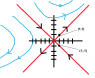# Classify the origin of the system and draw the phase portrait

• bjohnson2001

## Homework Statement

Draw the phase portrait and classify the origin of the system:

xdot = [1 2; 2 1]x

## Homework Equations

characteristic equation:

det(A-lambda*I) = 0

## The Attempt at a Solution

First find the eigenvalues and eigenvectors:

det(A-lambda*I) = (lambda+1)(lambda-3) = 0

we can see that the eigenvalues are:

lambda_1 = -1 and lambda_2 = 3

for lambda_1 = -1: 2*k1 + 2*k2 = 0
k1 = -k2

when k1 = 1, k2 = - 1 the related eigenvector is (1; -1)

for lambda_2 = 3: -2*k1 + 2*k2 = 0
k1 = k2

when k1 = 1, k2 = 1 the eigenvector is K2 = (1; 1)

since the matrix of coefficeints is a 2x2 matrix and since we found two linearly independent solutions,

the general solution of the system is:

X = c1*X1 + c2*X2 = c1*(1;-1)*exp(-t) + c2*(1; 1)*exp(t)
or
x = c1*exp(-t) + c2*exp(t)
y = -c1*exp(-t) + c2*exp(t)

We can classify the origin as neither a repeller nor an attractor.

Is this correct?

Also I feel like I need to provide more information when classifying the origin but I don't know what. For instance should I call the origin a saddle point because it has eigenvalues of different polarities?

#### Attachments

•phase plane.png
5.3 KB · Views: 489
The origin is a saddle. Also, I think you should draw a better phase portrait showing the flow in all four quadrants. Here's the code if you wish to do so in Mathematica but I don't think you can run it in Alpha:

StreamPlot[{x+2y,2x+y},{x,-2,2},{y,-2,2}]